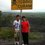# The Drag Force Equation

The drag force equation is $R = \frac{1}{2}D\rho Av^{2}$, where $v$ is the speed of an object, $A$ is the cross-sectional area of the object, $\rho$ is the density of the fluid, and $D$ is the drag coefficient. To go about proving the formula, i did this

From experimentation, it is evident that the drag force acting on an object is related to the objects velocity somehow. Hence I analysed an object moving with constant velocity in a fluid, and analysed the system to be the fluid. The power lost by the object would be

$P = \frac{W}{\Delta t}$

now if we look at a disk of air which has not yet been touched by the object. Let its velocity be 0. once the object hits that disk of air of mass m, it does work on it, and changes its kinetic energy to $\frac{1}{2}mv^{2}$

hence $P = \frac{W}{\Delta t} = \frac{\Delta K}{\Delta t} = \frac{mv^{2}}{2\Delta t}$

$\rho = \frac{m}{V}\Rightarrow m = \rho V$

$V = A\Delta r \Rightarrow m = \rho A\Delta r$, where $\Delta r$ is the distance that disk of air travelled

$v = \frac{\Delta r}{\Delta t} \Rightarrow \Delta r = v\Delta t$

hence, $m = \rho Av\Delta t$

subbing this back into the equation for power lost, we get

$P = \frac{\rho Av\Delta t v^{2}}{2\Delta t}$

$P = \frac{1}{2}\rho Av^{3}$

Since object is travelling at constant velocity, air resistance is constant, so power loss is constant. hence instantaneous power is equivelant to our average power equation above.

equation for instantaneous power is $P = \vec{F}\cdot\vec{v} = Fv$. Since the obect is in constant velocity, there is not net force so $F = R$. hence $P = Rv$

sub this back into our last power equation: $Rv = \frac{1}{2}\rho Av^{3}$

$R = \frac{1}{2}\rho Av^{2}$

my question is... where is the drag coefficient gone?6 years, 8 months ago

This discussion board is a place to discuss our Daily Challenges and the math and science related to those challenges. Explanations are more than just a solution — they should explain the steps and thinking strategies that you used to obtain the solution. Comments should further the discussion of math and science.

When posting on Brilliant:

• Use the emojis to react to an explanation, whether you're congratulating a job well done , or just really confused .
• Ask specific questions about the challenge or the steps in somebody's explanation. Well-posed questions can add a lot to the discussion, but posting "I don't understand!" doesn't help anyone.
• Try to contribute something new to the discussion, whether it is an extension, generalization or other idea related to the challenge.

MarkdownAppears as
*italics* or _italics_ italics
**bold** or __bold__ bold
- bulleted- list
• bulleted
• list
1. numbered2. list
1. numbered
2. list
Note: you must add a full line of space before and after lists for them to show up correctly
paragraph 1paragraph 2

paragraph 1

paragraph 2

[example link](https://brilliant.org)example link
> This is a quote
This is a quote
    # I indented these lines
# 4 spaces, and now they show
# up as a code block.

print "hello world"
# I indented these lines
# 4 spaces, and now they show
# up as a code block.

print "hello world"
MathAppears as
Remember to wrap math in $$ ... $$ or $ ... $ to ensure proper formatting.
2 \times 3 $2 \times 3$
2^{34} $2^{34}$
a_{i-1} $a_{i-1}$
\frac{2}{3} $\frac{2}{3}$
\sqrt{2} $\sqrt{2}$
\sum_{i=1}^3 $\sum_{i=1}^3$
\sin \theta $\sin \theta$
\boxed{123} $\boxed{123}$

Sort by:

We know that the drag coefficient is given by the object's shape (if it's flat, spherical, a cone shape etc.) and friction against its surfaces. In your proof you don't take these things into consideration, so you won't get the drag coefficient in your equation.

- 6 years, 8 months ago

Is not the objects shape taken to consideration by the $A$ in $R = \frac{1}{2}D\rho Av^{2}$

- 6 years, 8 months ago

Not really. $A$ is only the cross section area of the object in the direction in which it is travelling. For example, a cylinder, a sphere and a cone (all with the same base radius) will have the same value for $A$, but completely different values for $D$.

- 6 years, 8 months ago

What about a cylinder, a hemisphere and a cone, except the cone travels with is base hitting the air, the cylinder and hemisphere travels with its circular part hitting the air. In that case would it be same? Also if it isn't, how does shape affect air resistance

- 6 years, 8 months ago

If I interpret your question right (that all three objects travel with the flat, circular sides hitting the air), it would be more similar, but not completely. When the objects travel through the air, the air has to, in some way, move around the object. Its ability to do so is not only affected by the shape of the part directly hitting the air, but also by the sides and rear end of the object. Depending on the shape, there will be more or less turbulence behind the object, and turbulence will create drag. The key thing is that the air is a fluid which has to move around the object.

- 6 years, 8 months ago

Ok I get it thanks so much

- 6 years, 8 months ago